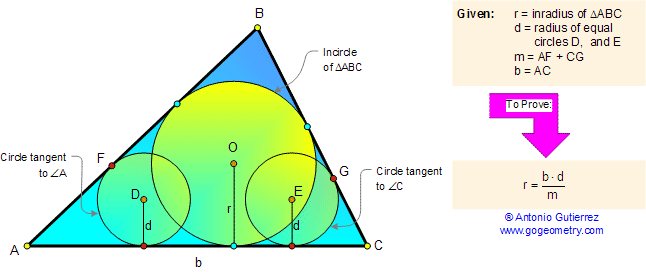< PREVIOUS PROBLEM  |  NEXT PROBLEM >

Problem 68. Triangle, Incircle, Inradius, Tangent. Level: High School, SAT Prep, College

 In the figure below, r is the inradius of a triangle ABC, d is the  radius of circles D and E tangent to the angles A and C respectively, and m = AF + CG. Prove that: $$r = \dfrac{b \cdot d}{m}$$.Home | Geometry | Search | Problems | 61-70 | Circle Tangent Line | Email | by Antonio Gutierrez Post or view a solution to the problem 68